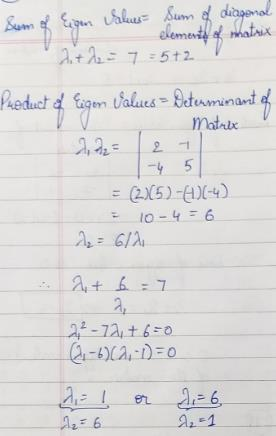Courses

# Test: Eigenvalues & Eigenvectors - 2

## 25 Questions MCQ Test Topicwise Question Bank for Civil Engineering | Test: Eigenvalues & Eigenvectors - 2

Description
This mock test of Test: Eigenvalues & Eigenvectors - 2 for GATE helps you for every GATE entrance exam. This contains 25 Multiple Choice Questions for GATE Test: Eigenvalues & Eigenvectors - 2 (mcq) to study with solutions a complete question bank. The solved questions answers in this Test: Eigenvalues & Eigenvectors - 2 quiz give you a good mix of easy questions and tough questions. GATE students definitely take this Test: Eigenvalues & Eigenvectors - 2 exercise for a better result in the exam. You can find other Test: Eigenvalues & Eigenvectors - 2 extra questions, long questions & short questions for GATE on EduRev as well by searching above.
QUESTION: 1

### For the matrix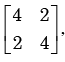the eigen value corresponding to the eigenvector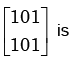Solution: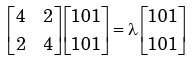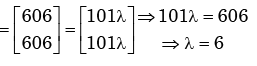*Multiple options can be correct
QUESTION: 2

### The eigen values of the matrix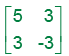Solution: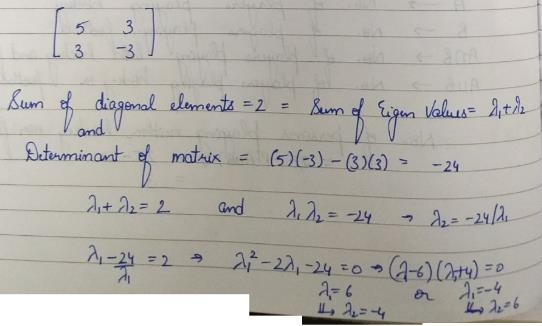QUESTION: 3

### For the matrix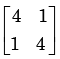the eigen value are

Solution: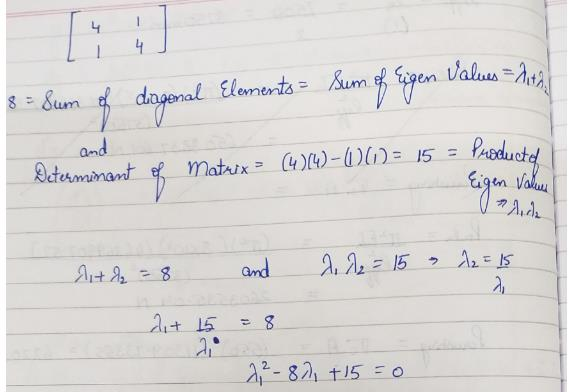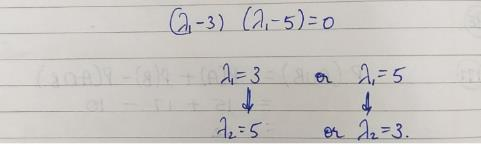QUESTION: 4

For which value of x will the matrix given below become singular?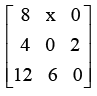Solution:

Let the given matrix be A.  A is singular.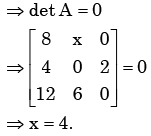QUESTION: 5

If a square matrix A is real and symmetric, then the eigenvaluesn

Solution: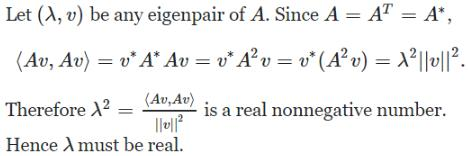QUESTION: 6

The matrix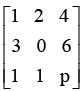has one eigenvalue equal to 3. The sum of the other two eigenvalues is

Solution:

Let the given matrix be  A.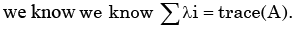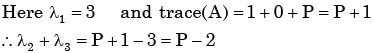QUESTION: 7

For a matrix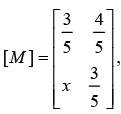the transpose of the matrix is equal to the inverse of the  matrix,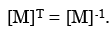The value of x is given by

Solution: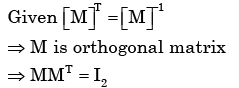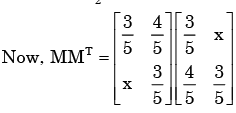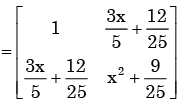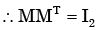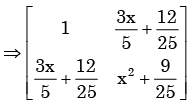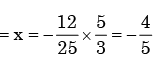QUESTION: 8

The eigen values of the matrix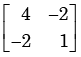Solution: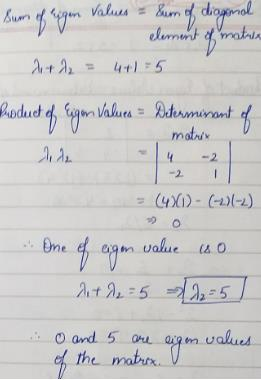QUESTION: 9

For a given matrix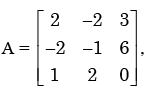one of the eigenvalues is 3. The other two eigenvalues are

Solution: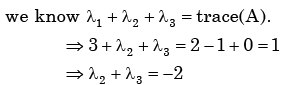QUESTION: 10

The Eigen values of the matrix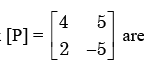Solution: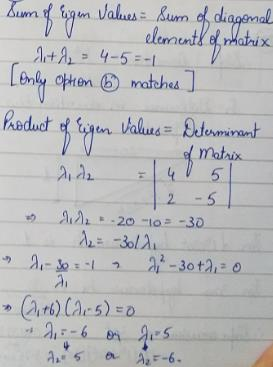QUESTION: 11

In the matrix equation Px = q which of the following is a necessary condition for the existence of at least one solution for the unknown vector x:

Solution:
QUESTION: 12

If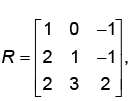then top row of R-1 is

Solution: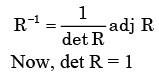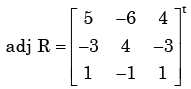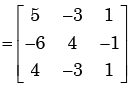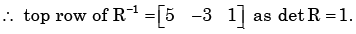QUESTION: 13

Cayley - Hamiltion Theorem states that square matrix satisfies its own characteristic equation, Consider a matrix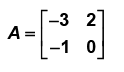A satisfies the relation

Solution:

Characteristic equation of A is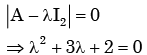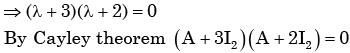QUESTION: 14

The characteristic equation of a (3×3) matrix P is defined as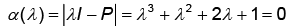If I denote identity matrix, then the inverse of matrix P will be

Solution:

Given ch. equof A is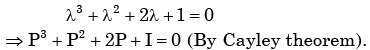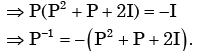QUESTION: 15

Let P be a 2×2 real orthogonal matrix and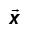s a real vector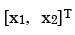with length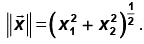Then which one of the following statements is correct?

Solution: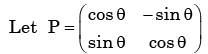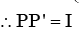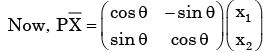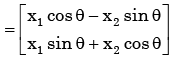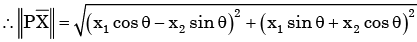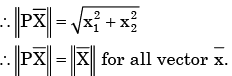QUESTION: 16

An eigenvector of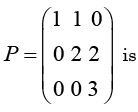Solution:

Eigen values of P are 1,2,3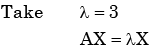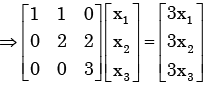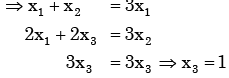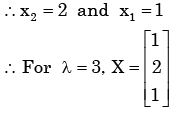QUESTION: 17

Let A be an n × n real matrix such that A2 = I and y = be an n – dimensional vector.  Then the linear system of equations Ax = y has

Solution: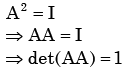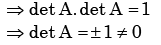By Cramer’s rule AX =y has unique solution.

QUESTION: 18

A real n × n matrix A = {aij} is defined as follows:

aij = i = 0, if

i  = j, otherwise

The summation of all n eigen values of A is

Solution:

It’s a diagonal marix diagonal contain’s  n elements 1,2,----,n.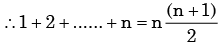As diagonal elements are eigen valves.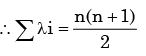QUESTION: 19

The following system of equations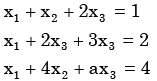has a unique solution. The only possible value(s) for a is/are

Solution: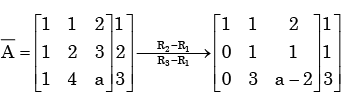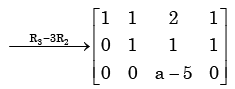System has unique Soln if  rank (A) = rank ( A ) = 3 . It is possible if a ≠ 5.

QUESTION: 20

The eigenvalues of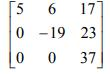are

Solution:

The eigenvalues of an upper triangular matrix are simply the diagonal entries of the matrix. Hence 5, -19, and 37 are the eigenvalues of the matrix. Alternately, look at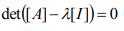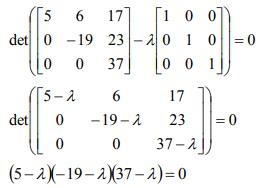Then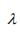= 5,-19,37 are the roots of the equation; and hence, the eigenvalues of [A].

QUESTION: 21

The number of different n × n symmetric matrices with each element being either 0 or 1 is: (Note : power (2, x) is same as 2x)

Solution:

In a symmetric matrix, the lower triangle must be the minor image of upper triangle using the diagonal as mirror. Diagonal elements may be anything. Therefore, when we are counting symmetric matrices we count how many ways are there to fill the upper triangle and diagonal elements. Since the first row has n elements, second (n – 1) elements, third row (n – 2) elements and so on upto last row, one element.  Total number of elements in diagonal + upper triangle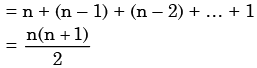Now, each one of these elements can be either 0 or 1. So that number of ways we can fill these elements is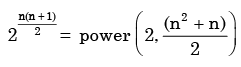Since there is no choice for lower triangle elements the answer is power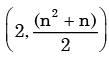which
is choice (c).

QUESTION: 22

In an M × N matrix such that all non-zero entries are covered in a rows and b column. Then the maximum number of non-zero entries, such that no two are on the same row or column, is

Solution:

Suppose a < b, for example let a = 3, b= 5, then we can put non-zero entries only in 3 rows and 5 columns. So suppose we put non-zero entries in any 3 rows in 3 different columns. Now we can’t put any other non-zero entry anywhere in matrix, because if we put it in some other row, then we will have 4 rows containing non-zeros, if we put it in one of those 3 rows, then we will have more than one non-zero entry in one row, which is not allowed.

So we can fill only “a” non-zero entries if a < b, similarly if b < a, we can put only “b” non-zero entries. So answer is ≤min(a,b), because whatever is less between a and b, we can put atmost that many non-zero entries.

QUESTION: 23

Consider the following system of equation in three real variables x1, x2 and x3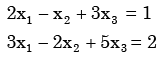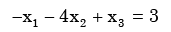This system of equations has

Solution: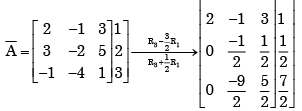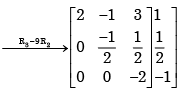∴ Rank (A)= Rank ( A ) = 3

QUESTION: 24

How many of the following matrics have an eigenvalue 1?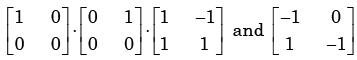Solution: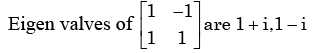Rest given matrix are triangular matrix. so diagonal elements are the eigen  values.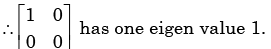QUESTION: 25

What are the eigen values of the following 2 × 2 matrix?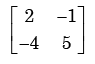Solution: